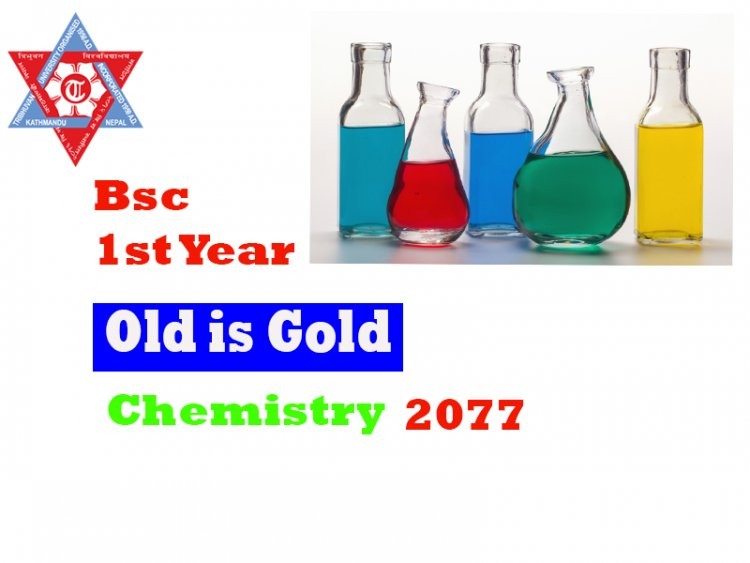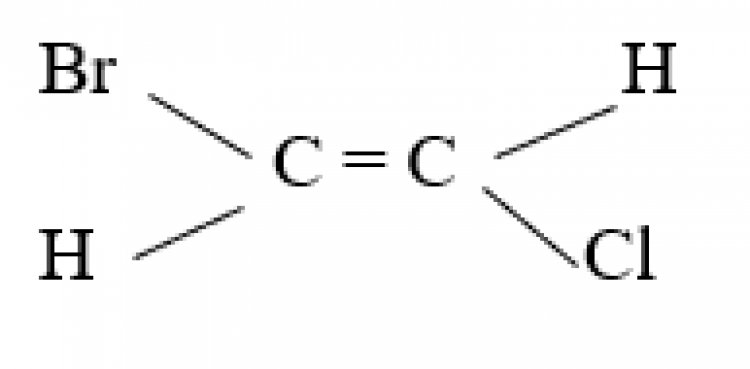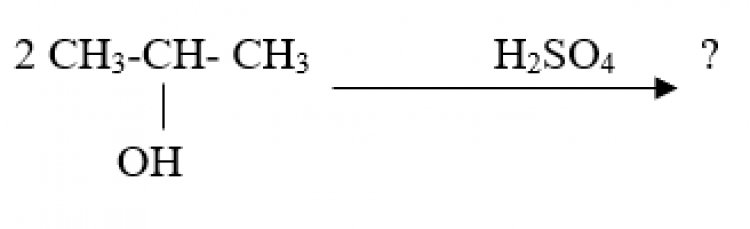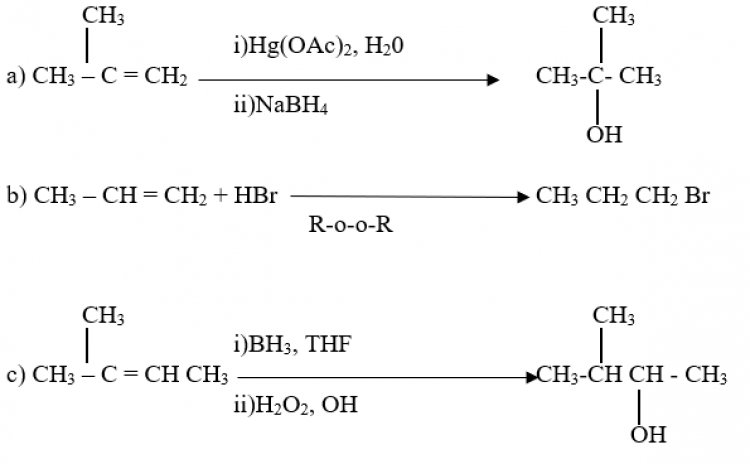# Bsc I Year Chemistry Question-2077

## Bsc I Year Chemistry Question-2077Use separate answer-book for each -group.

The comprehensive question of each group is compulsory.

Attempt SIX questions of Short Answer Questions of each Group.

(New Course)

GROUP "A" (INORGANIC)

1. Comprehensive question

What are the short comings of Bohr's theory of atomic structure? How are time short comings addressed in the modern theory?  [4+5]

OR

What is the difference between electron affinity and electro negatively? How does the electronegativity of an element differ along the period and along the group? [5+4]

2. Short Answer questions - 6x4=24

2.1. What is meant by Heisenberg’ S uncertainty principle? Explain.

2.2. What is meant by nuclear fission and nuclear fusion?

2.3. Draw and briefly explain ‘the structure of TiO2?

2.4. What are the main postulates of VSEPR theory?

2.5. What is meant by gravimetric analysis? Point out any two requirements that should be met for a successful gravimetric analysis.

2.6. Product the bond order in Ne2, O2, CO and NO using molecular orbital theory.

2.7. What is meant by Born-Lande equation? For what purpose this equation is used?

2.8. Give examples of hard acid, soft acids, hard bases and soft bases. Also explain the bases classifying such species as hard and soft.

2.9. What is meant by percentage ionic character of a covalent bond? How is this estimated?

GROUP "B" (ORGANIC)

3. Comprehensive Question

Give the mechanism of E1 and E2 reaction. A primary alkyl halide (X) upon dehydrohalogenation gives an alkene (Y) which upon ozonolysis yields a mixture of acetaldehyde and formaldehyde. Identify X and Y giving mechanism. [4+5]

OR

Define the term configuration. The specification of configuration (R or S) is based on a set of sequence rules. Justify this statement with appropriate examples. [2+7]

4. Short Answer questions - 6x4=24

4.1. Account for the fact that o-nitrophenol is more volatile than p-nitrophenol.

4.2. How would you prepare alkane of higher carbon number than a starting compound using lithium dialkyl copper? Give the mechanism.

4.3. Account for the fact that the SN2 reaction proceeds with the complete inversion of configuration.

4.4. What do you mean by E and Z configuration? Assign the following compound as E or Z.4.5. What do you mean by plane polarized light and specific rotation? Explain these terms with appropriate illustration.

4.6. Give the mechanism and product of the following conversion.4.7. Discuss the factors affecting in the reactivity of SN1 and SN2? reaction.

4.8. What product will be farmed when propyne is hydrated with sulphuric acid in presence of mercuric sulphate? Explain it with giving mechanism.

4.9. Draw the labelled energy profile diagram for one and two step exothermic reaction.

GROUP "C" (PHYSICAL)

5. Comprehensive question.

What do you mean by r m s velocity of gas molecules? Deduce the following relation; RTc / PcVc = 8/3, where the symbols have their usual meanings.

2 moles of CO2, at 27°C is filled in a 5 L flask. Calculate the pressure using the following:

(i) Ideal gas equation and (ii) van der Waals equation.

For CO2, a= 3.6 atm L2 mole-2, b= 4.28x10-2 L mol-1 and R= 0.082 L atm K-1 mol-1. [2+3+5]

OR

Deduce an expression for the degree of hydrolysis of a salt of weak acid and strong base.

The pH of a solution of NH4Cl is 4.84. Calculate the molarity of the solution and degree of hydrolysis of the salt in the given solution. Given, Kb = 1.8x10° [5+5]

6. Short Answer questions - 6x4=24

6.1 What are the factors that affect the collision frequency? Define collision diameter.

6.2. Show your acquaintance with "aqueous tension" and “surface tension”.

6.3. The equilibrium constant for the esterification of ethanol and ethanoic acid is 4 at 298K. If 60 g of ethanol and 60 g of ethanoic acid were mixed, how much ester would be formed at the same temperature?

6.4. Define colligative property and show that relative lowering of vapour pressure of a dilute solution is a colligative property.

6.5. Define isotonic, hypertonic and hypotonic solutions. 1.5% cane sugar solution is found to be isotonic with x M urea solution at the same temperature. Find x.

6.6. Show that the half-life period of a second order reaction is inversely proportional to the initial concentration of the reactant.

6.7. Define half-life of a reaction. How can you use the value of half-life to determine the order of a reaction?

6.8. Derive Kirchhoff’s equation and mention its applications.

6.9. Define bond enthalpy. Calculate the enthalpy of the formation of water vapour. Given bond enthalpies of H-H, H-O and O=O bonds as 435,464 and 499KJ mol-1 respectively.

(Old Course)

The comprehensive question of each group is compulsory.

Attempt SIX questions of Short Answer Questions of each Group.

GROUP "A" (INORGANIC)

1. Comprehensive Question

(a) What is meant by the term" Dual nature of electron"? Derive de-Broglie's equation.

(b) Explain Heisenberg's uncertainty principle. Show that this principle is valid only for a small particle and not for large objects. [4+5]

OR

What is lattice energy? Derive the Born-Lande equation for lattice energy. [1+8]

2. Short Answer questions (attempt any six) 6x4=24

2.1 State Pauli's exclusion principle and show that in a given shell, there can be only two s-and six p-electrons.

2.2 Explain the terms: (a) Binding energy and (b) Mass defects.

2.3 Give the significance and limitations of long form of periodic table.

2.4 What are the parameters on which each of the following electronegativity scales is based?

(a) Pauling's scale (b) Allred and Rochow scale

2.5 Based on VSEPR model predict the most likely structure of CIF3

2.6 Using molecular orbital theory determine the bond order of O2 molecule. Is it paramagnetic? How are stability related to the bond length and bond order?

2.7 Explain the terms: conductors, insulators and semiconductors.

2.8 What is HSAB principle. Explain why [Ag (CN)2]- is very stable but [AgCl2]- is not stable.

2.9 Why is NH4Cl used along with NH4OH in the qualitative analysis of basic radicals by wet way in Gr III A?

GROUP "B" (ORGANIC)

3. Comprehensive question

Give mechanism of the following reactions; [3+3+3]OR,

What is the cause of lesser reactivity of alkanes? Explain why the most stable conformer of n-butane is the anti, synthesize n-hexane and 2-methylpentane using Corey - House synthesis. [2+3+4]

4.1. What do you understand by the term "Resonance"? How does it contribute towards the stability of the benzene?

4.2. Explain with giving example the ease of abstraction of hydrogen in alkane.

4.3. Draw a structural formula for an optically active compound with the molecular formula:

a) C4H100  b) C5H11Cl c) C4H5(OH)2 d) C6H12

4.4. Give reasons for the following observations

a)SN2 reactions proceed with complete inversion of configurations

b) The greater the dispersal of the positive charge, the greater is the stability of the carbocation.

4.5. Treating 3-methyl - 2butanol with HBr yields 2-bromo-2-methyl butane as the sole product, outline mechanism for the reaction.

4.6. Draw structures and give (E) - (Z) designations for the isomers of:

a) 3 - chloro - 1,4 - hexadiene

b) 2 - bromo - 4 - chloro - 2 hexen - 5yne

4.7. Write equations for the following reactions:

a) 2 - pentyne + H2 (1 mol, Lindlar's catalyst) → ?

b) 3 - Hexyne + Cl2 (2 mol) → ?

c) Propyne + sodium amide in liquid ammonia → ?

d) 1 - Butyne + H2O (H+, HgSo4 catalyst) → ?

4.8. Write a concise note on Phose transfer catalysis.

4.9. What product would you expect from SN2 reaction of 1 - bromobutane with each of the following?

a)NaI    b. KOH   c) H-C ≡ CLi   d)NH3

GROUP "C" (PHYSICAL)

5. Comprehensive questions.

Derive the van der Waal's equation considering the volume and pressure corrections for real gas. Write the dimensional formula of the van der Waal constants.

Calculate the root mean square velocity of oxygen molecule at NTP. [4+2+4]

OR

Define the specific and molar heat capacities? Derive equations for molar heat capacity at constant pressure and volume. Calculate the Kp and Kc ratio at 298 K for the reaction of:

N2(g)+3H2(g) ⇔ 2NH3(g).           [1+5+4]

6. Short Answer Questions. 6x4 24

6.1. Calculate the average Kinetic energy of one mole of nitrogen gas at 300 K (R = 8.314 JK-1 mol-1

6.2. Comparatively describe the principles of Linde’s and Claud's methods of Liquefaction of air. 6.3. Classify the solid based on bond types and write their properties.

6.4. Ethanol and ethanoic acid are mixed together in 1:1 molar ratio at 25°C and 50% of both reactants converted into ester. Calculate the equilibrium constant for the transformation.

6.5. Write a short note on the theory of acid base indicator.

6.6. What are colligative properties? Explain how the osmotic pressure and elevation of boiling point are used to determine the molecular weight of solute.

6.7. Point out the differences between lyophilic and lyophobic colloids.

6.8. Define the electrophoresis and electro-osmosis. Point out their applications.

6.9. What is buffer solution? Describe the working principles of acidic and basic buffer solutions.